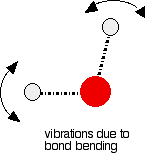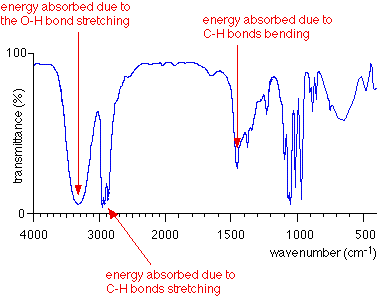# IR Spectroscopy Background

$$\newcommand{\vecs}{\overset { \rightharpoonup} {\mathbf{#1}} }$$ $$\newcommand{\vecd}{\overset{-\!-\!\rightharpoonup}{\vphantom{a}\smash {#1}}}$$$$\newcommand{\id}{\mathrm{id}}$$ $$\newcommand{\Span}{\mathrm{span}}$$ $$\newcommand{\kernel}{\mathrm{null}\,}$$ $$\newcommand{\range}{\mathrm{range}\,}$$ $$\newcommand{\RealPart}{\mathrm{Re}}$$ $$\newcommand{\ImaginaryPart}{\mathrm{Im}}$$ $$\newcommand{\Argument}{\mathrm{Arg}}$$ $$\newcommand{\norm}{\| #1 \|}$$ $$\newcommand{\inner}{\langle #1, #2 \rangle}$$ $$\newcommand{\Span}{\mathrm{span}}$$ $$\newcommand{\id}{\mathrm{id}}$$ $$\newcommand{\Span}{\mathrm{span}}$$ $$\newcommand{\kernel}{\mathrm{null}\,}$$ $$\newcommand{\range}{\mathrm{range}\,}$$ $$\newcommand{\RealPart}{\mathrm{Re}}$$ $$\newcommand{\ImaginaryPart}{\mathrm{Im}}$$ $$\newcommand{\Argument}{\mathrm{Arg}}$$ $$\newcommand{\norm}{\| #1 \|}$$ $$\newcommand{\inner}{\langle #1, #2 \rangle}$$ $$\newcommand{\Span}{\mathrm{span}}$$$$\newcommand{\AA}{\unicode[.8,0]{x212B}}$$

This page describes what an infra-red spectrum is and how it arises from bond vibrations within organic molecules.

## How an infra-red spectrum is produced

You probably know that visible light is made up of a continuous range of different electromagnetic frequencies - each frequency can be seen as a different color. Infra-red radiation also consists of a continuous range of frequencies - it so happens that our eyes can't detect them.

If you shine a range of infra-red frequencies one at a time through a sample of an organic compound, you find that some frequencies get absorbed by the compound. A detector on the other side of the compound would show that some frequencies pass through the compound with almost no loss, but other frequencies are strongly absorbed.

How much of a particular frequency gets through the compound is measured as percentage transmittance. A percentage transmittance of 100 would mean that all of that frequency passed straight through the compound without any being absorbed. In practice, that never happens - there is always some small loss, giving a transmittance of perhaps 95% as the best you can achieve. A transmittance of only 5% would mean that nearly all of that particular frequency is absorbed by the compound. A very high absorption of this sort tells you important things about the bonds in the compound.

## What an infra-red spectrum looks like

A graph is produced showing how the percentage transmittance varies with the frequency of the infra-red radiation.Notice that an unusual measure of frequency is used on the horizontal axis. Wavenumber is defined like this:Similarly, don't worry about the change of scale half-way across the horizontal axis. You will find infra-red spectra where the scale is consistent all the way across, infra-red spectra where the scale changes at around 2000 cm-1, and very occasionally where the scale changes again at around 1000 cm-1. As you will see when we look at how to interpret infra-red spectra, this does not cause any problems - you simply need to be careful reading the horizontal scale.

## What causes some frequencies to be absorbed?

Each frequency of light (including infra-red) has a certain energy. If a particular frequency is being absorbed as it passes through the compound being investigated, it must mean that its energy is being transferred to the compound.

Energies in infra-red radiation correspond to the energies involved in bond vibrations.

## Bond stretching

In covalent bonds, atoms aren't joined by rigid links - the two atoms are held together because both nuclei are attracted to the same pair of electrons. The two nuclei can vibrate backwards and forwards - towards and away from each other - around an average position.

The diagram shows the stretching that happens in a carbon-oxygen single bond. There will, of course, be other atoms attached to both the carbon and the oxygen. For example, it could be the carbon-oxygen bond in methanol, CH3OH.The energy involved in this vibration depends on things like the length of the bond and the mass of the atoms at either end. That means that each different bond will vibrate in a different way, involving different amounts of energy.

Bonds are vibrating all the time, but if you shine exactly the right amount of energy on a bond, you can kick it into a higher state of vibration. The amount of energy it needs to do this will vary from bond to bond, and so each different bond will absorb a different frequency (and hence energy) of infra-red radiation.

## Bond bending

As well as stretching, bonds can also bend. The diagram shows the bending of the bonds in a water molecule. The effect of this, of course, is that the bond angle between the two hydrogen-oxygen bonds fluctuates slightly around its average value. Imagine a lab model of a water molecule where the atoms are joined together with springs. These bending vibrations are what you would see if you shook the model gently.Again, bonds will be vibrating like this all the time and, again, if you shine exactly the right amount of energy on the bond, you can kick it into a higher state of vibration. Since the energies involved with the bending will be different for each kind of bond, each different bond will absorb a different frequency of infra-red radiation in order to make this jump from one state to a higher one.

## Tying all this together

Look again at the infra-red spectrum of propan-1-ol, CH3CH2CH2OH:In the diagram, three sample absorptions are picked out to show you the bond vibrations which produced them. Notice that bond stretching and bending produce different troughs in the spectrum.

## Contributor

Jim Clark (Chemguide.co.uk)

This page titled IR Spectroscopy Background is shared under a CC BY-NC 4.0 license and was authored, remixed, and/or curated by Jim Clark.